# RS Aggarwal Solutions for Class 10 Maths Chapter 9 Constructions Exercise 9A

R S Aggarwal Solutions for Class 10 Maths Chapter 9 Constructions, contains solutions for exercise 9A questions. This exercise is all about division of a line segment in a given ratio using different methods and construction of a triangle similar to a given triangle as per given scale factor. Students can download the R S Aggarwal Solutions of Class 10 and clear their doubts on any Maths topic.

## Download PDF of RS Aggarwal Solutions for Class 10 Maths Chapter 9 Constructions Exercise 9A Page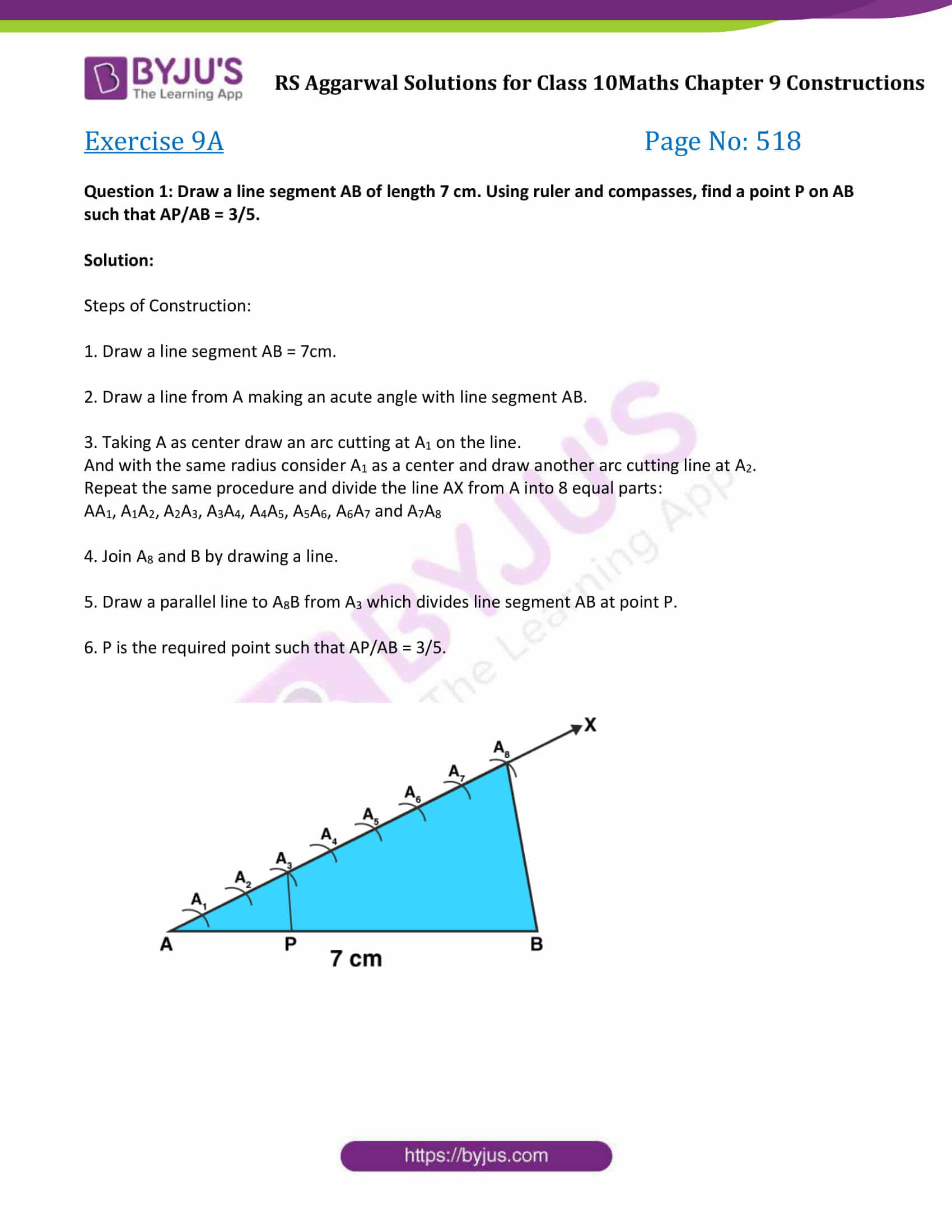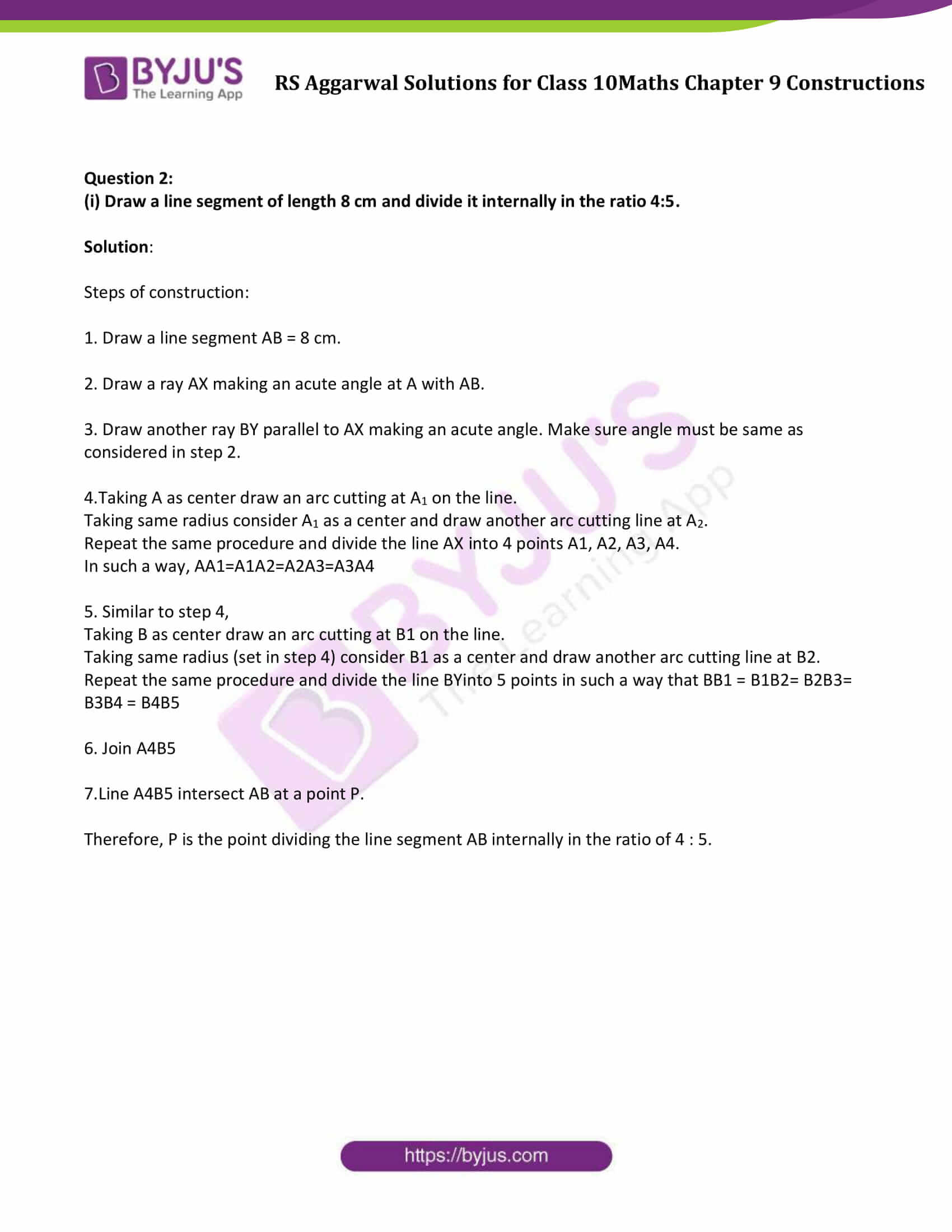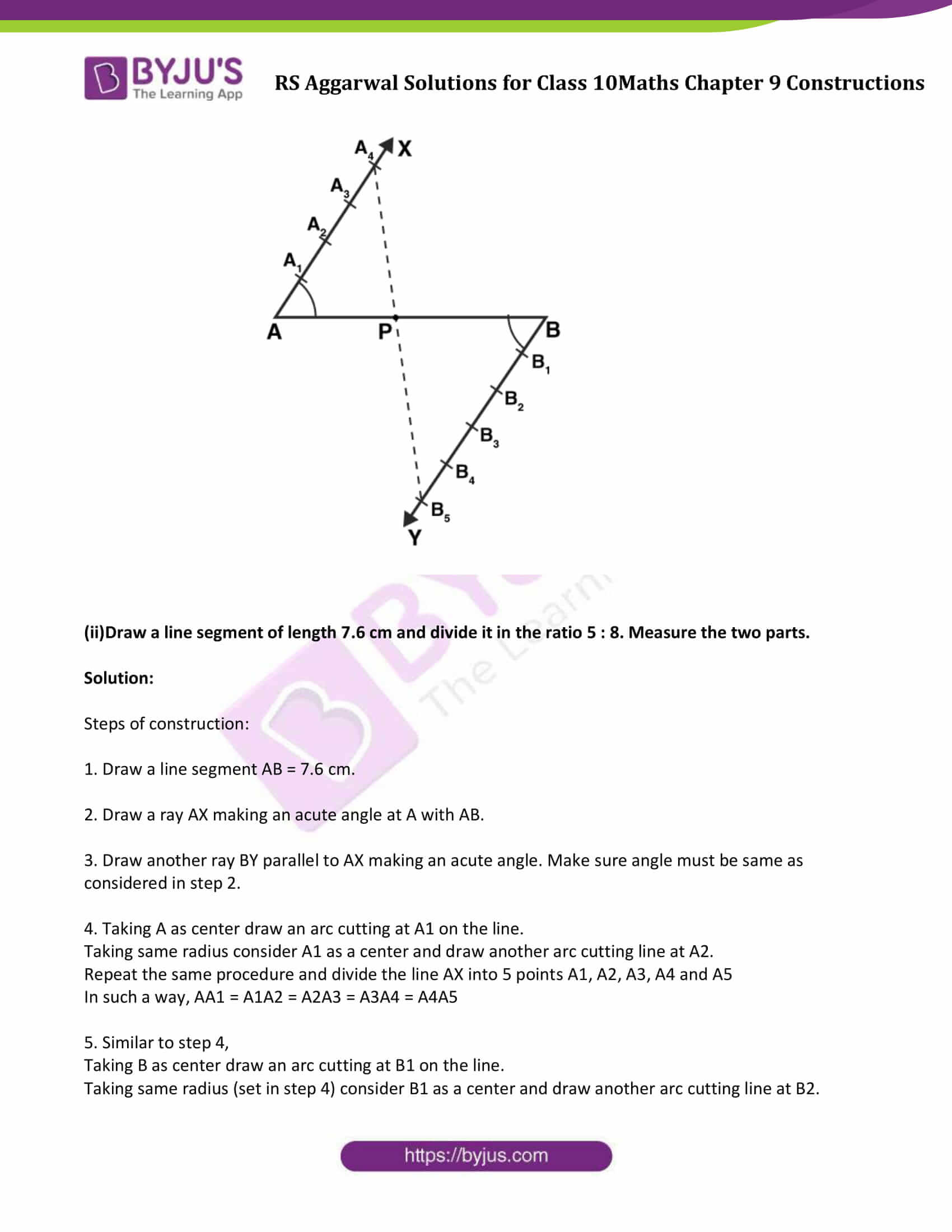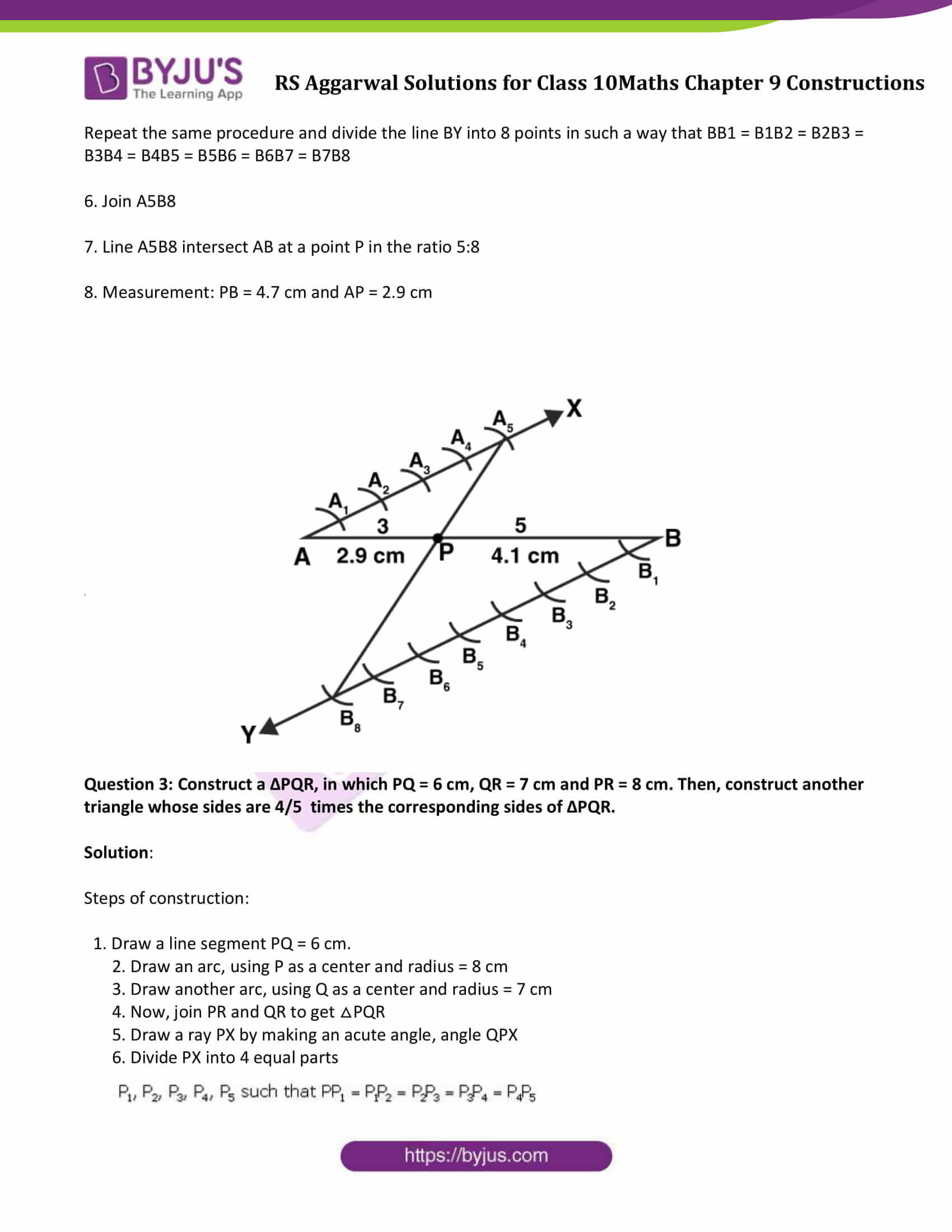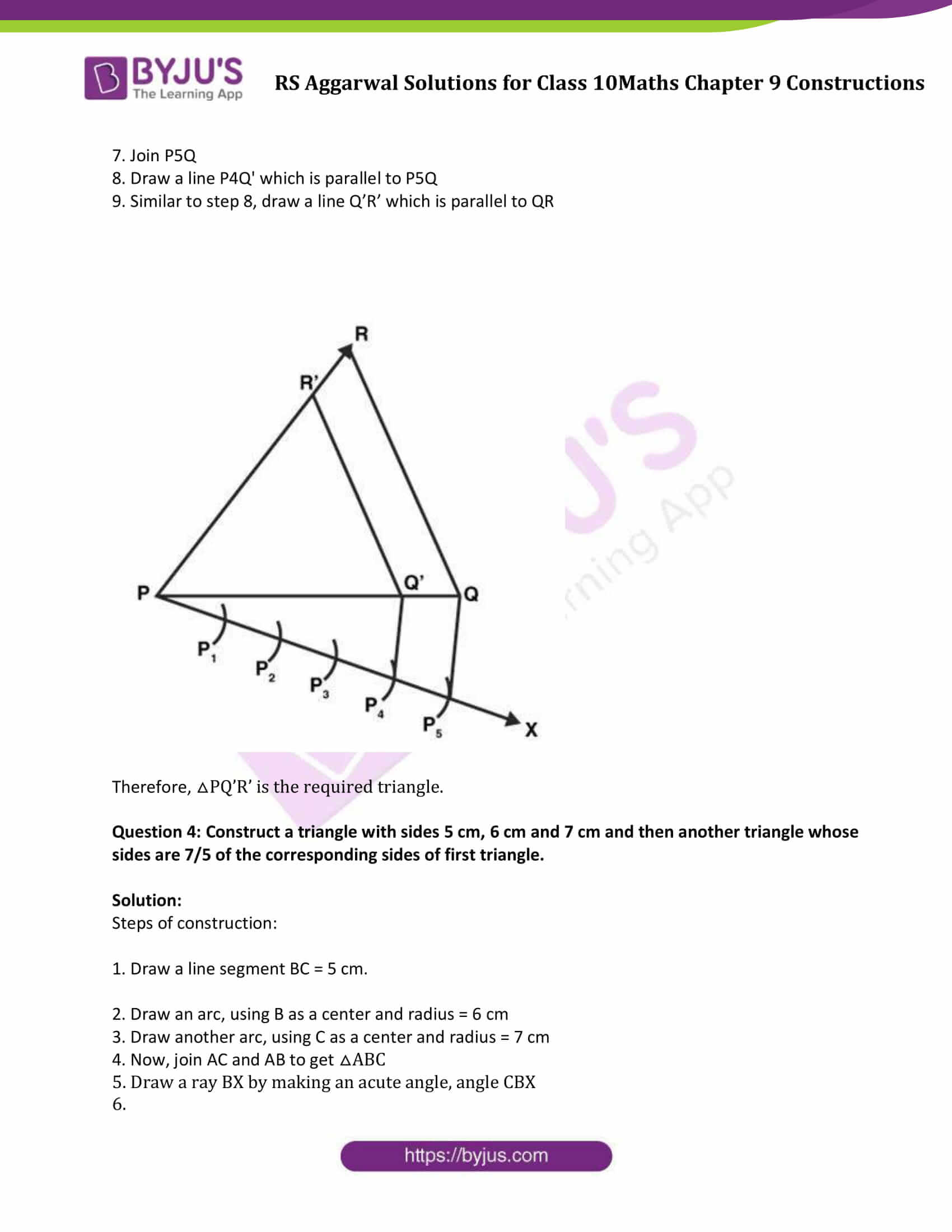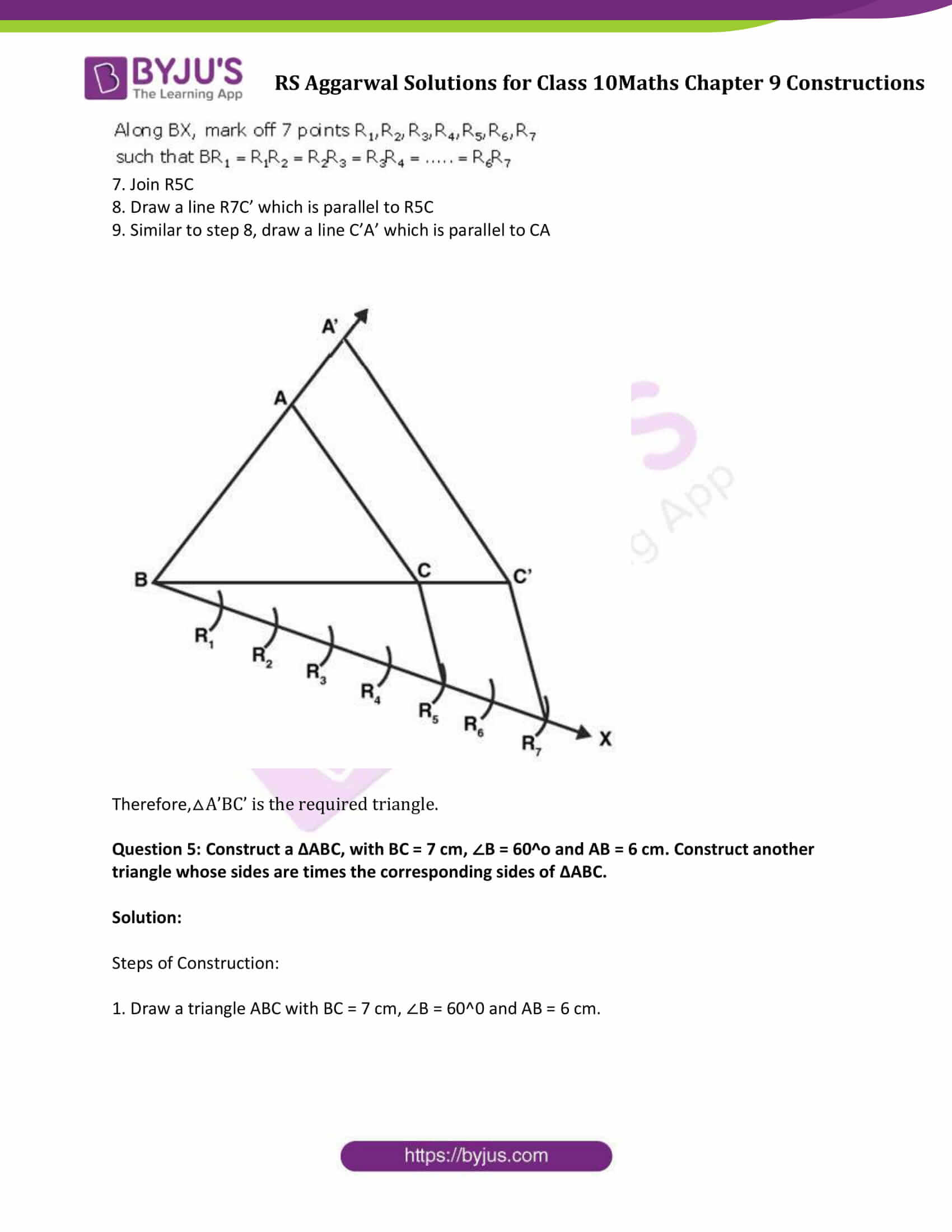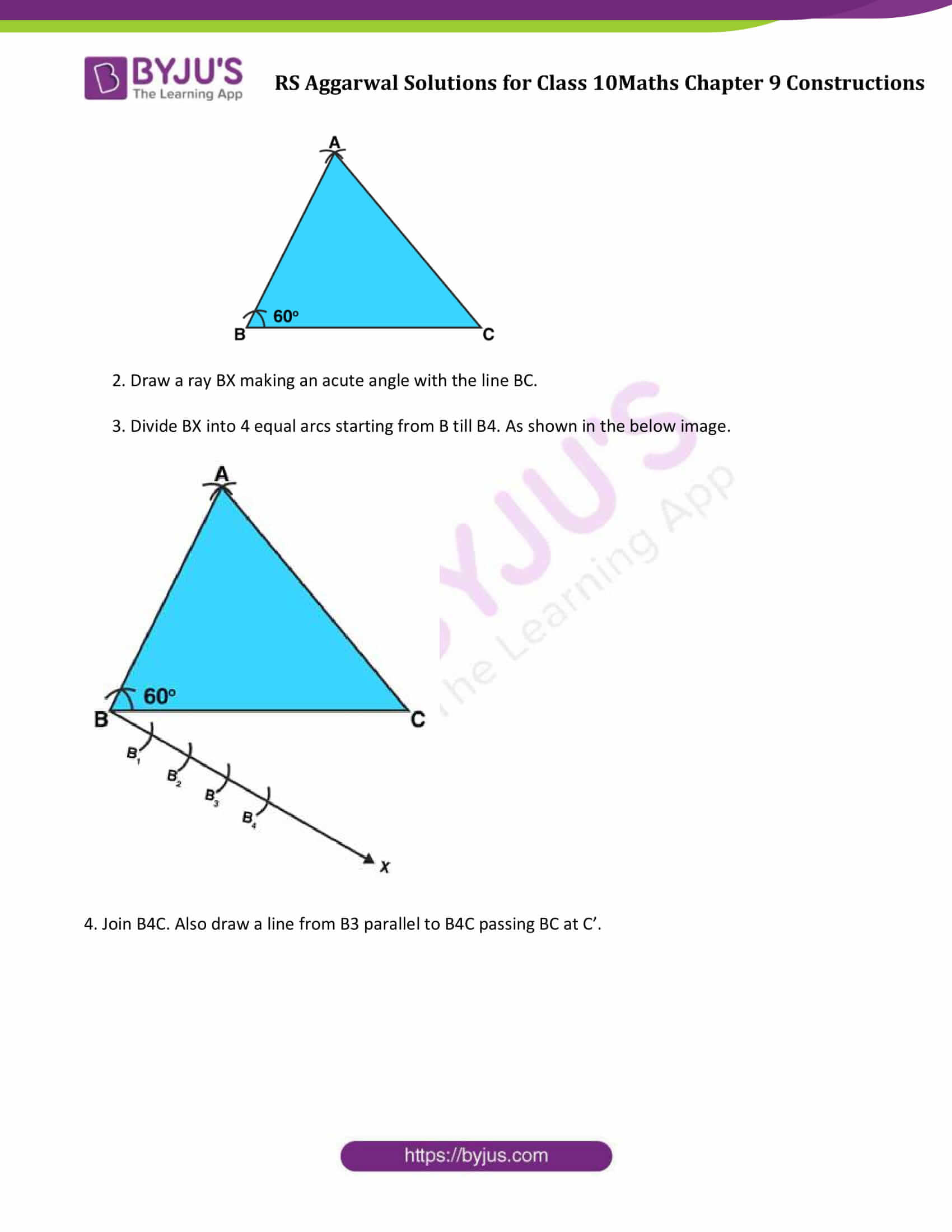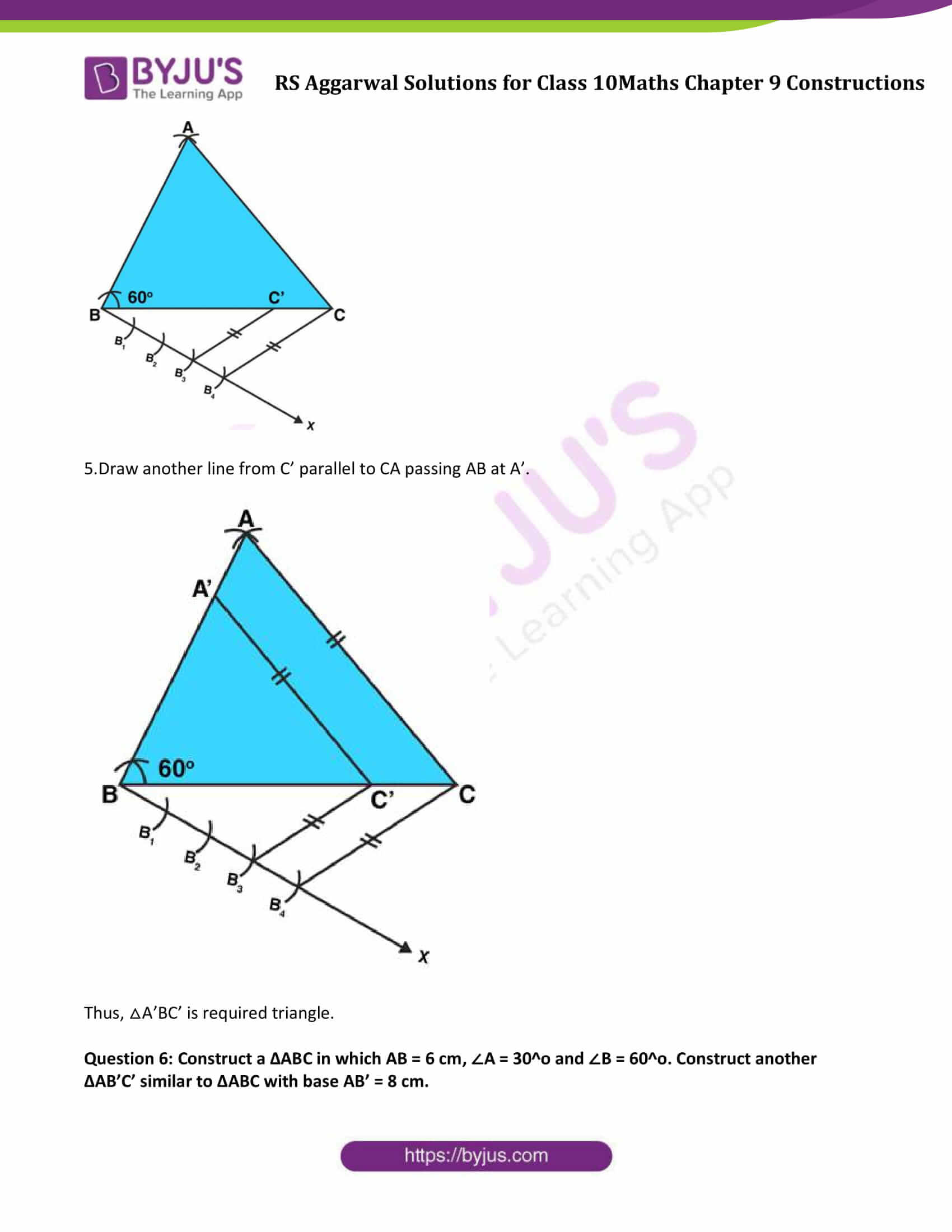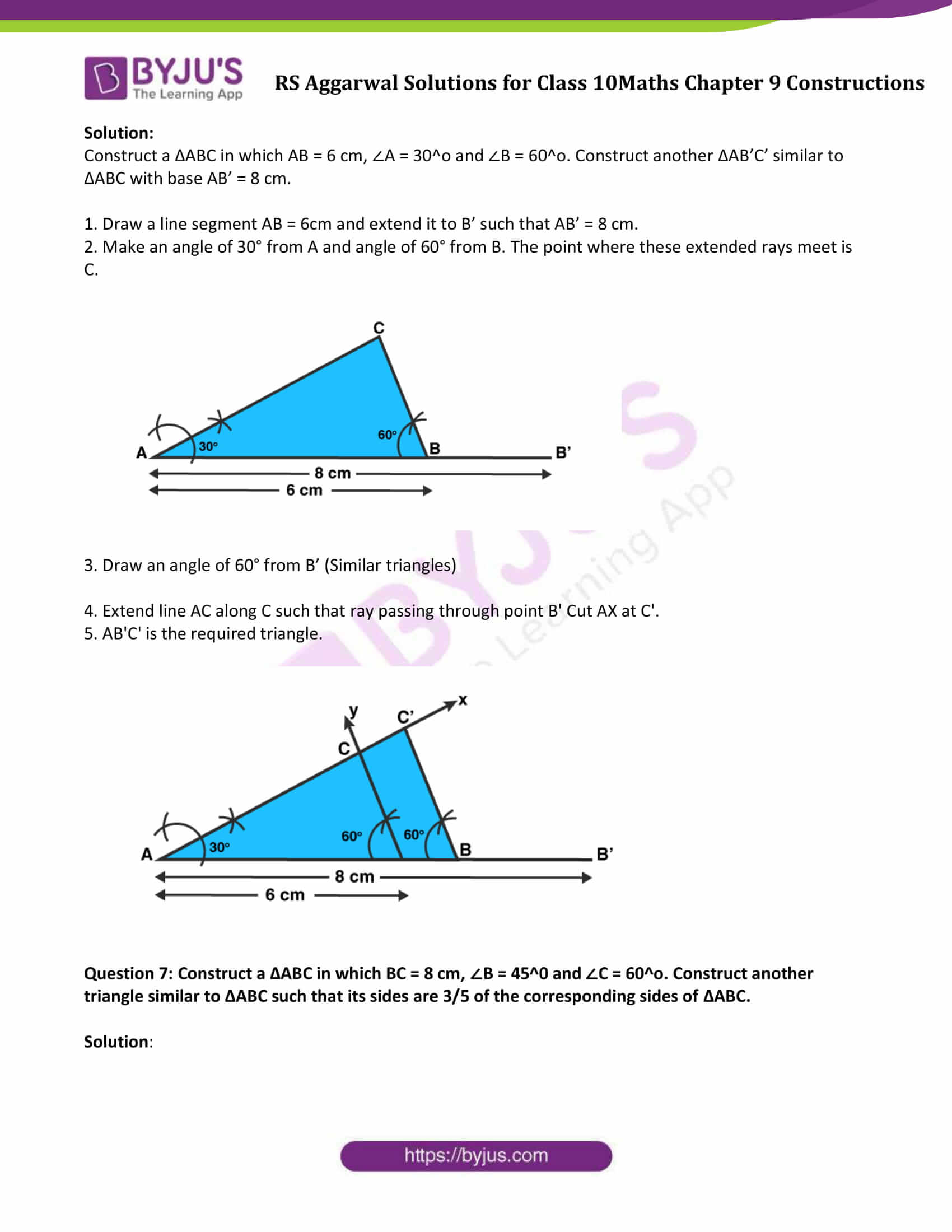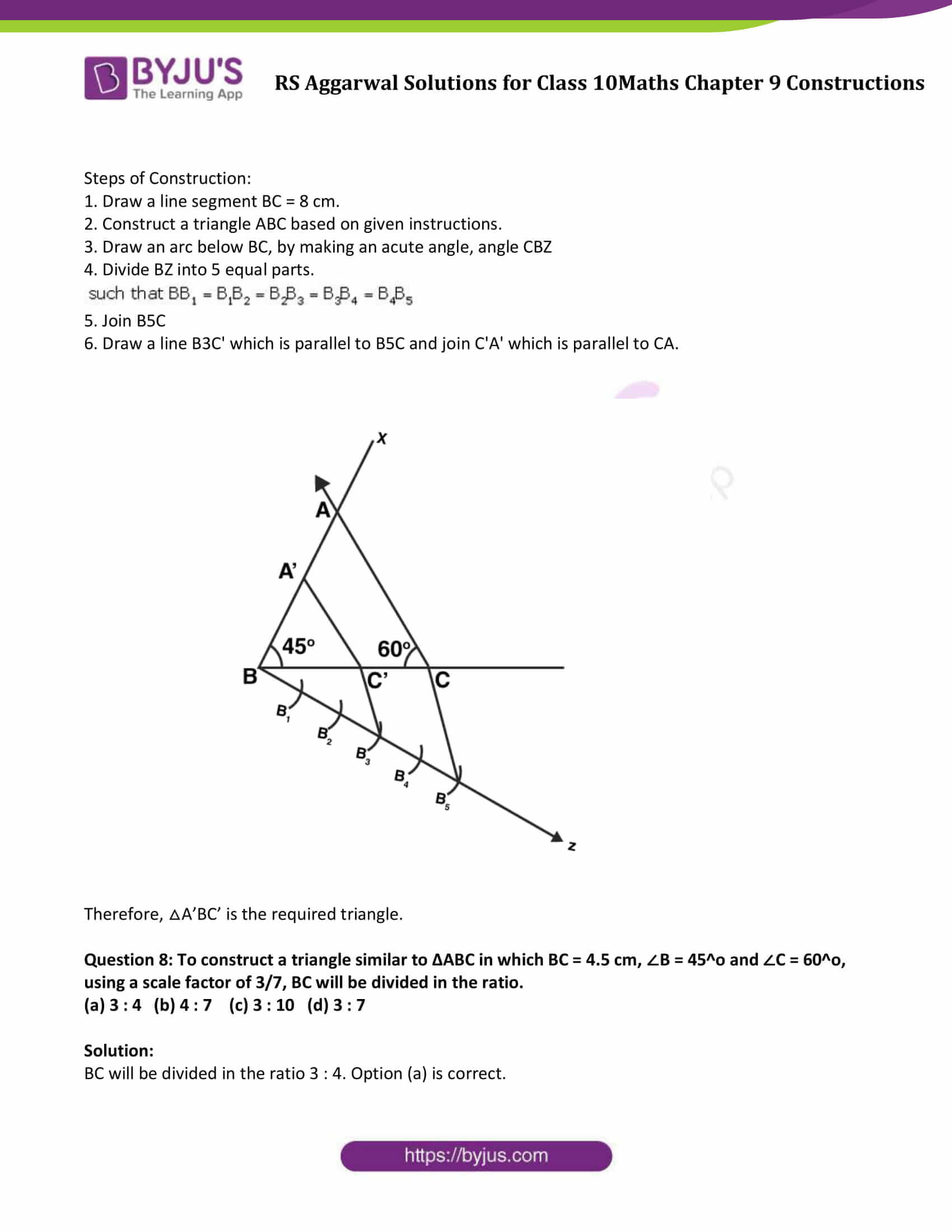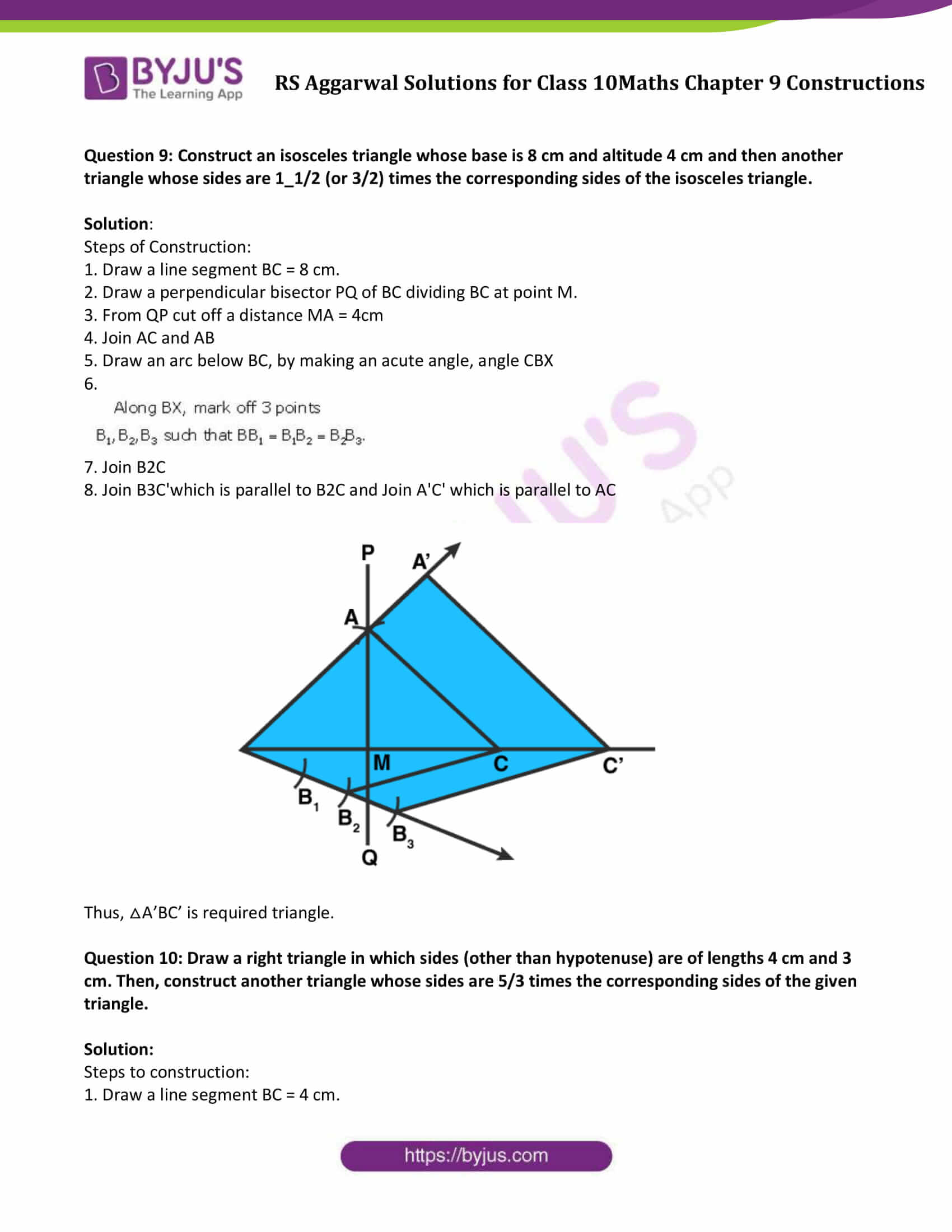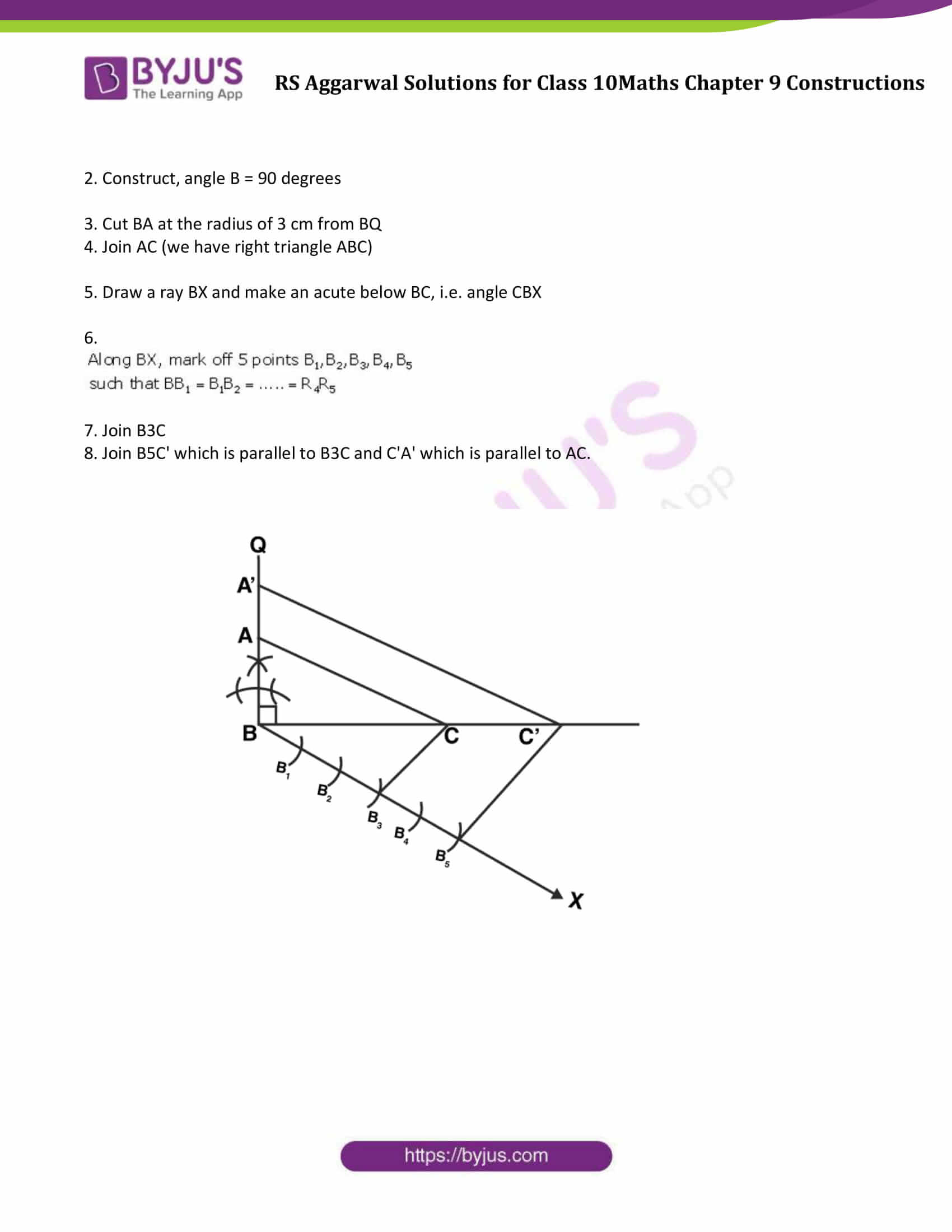### Access other exercise solutions of Class 10 Maths Chapter 9 Constructions

Exercise 9B Solutions

## Exercise 9A

Question 1: Draw a line segment AB of length 7 cm. Using ruler and compasses, find a point P on AB such that AP/AB = 3/5.

Solution:

Steps of Construction:

1. Draw a line segment AB = 7cm.

2. Draw a line from A making an acute angle with line segment AB.

3. Taking A as center draw an arc cutting at A1 on the line.

And with the same radius consider A1 as a center and draw another arc cutting line at A2.

Repeat the same procedure and divide the line AX from A into 8 equal parts:

AA1, A1A2, A2A3, A3A4, A4A5, A5A6, A6A7 and A7A8

4. Join A8 and B by drawing a line.

5. Draw a parallel line to A8B from A3 which divides line segment AB at point P.

6. P is the required point such that AP/AB = 3/5.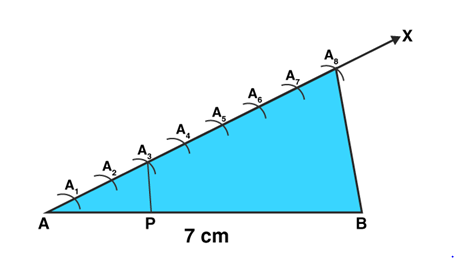Question 2:

(i) Draw a line segment of length 8 cm and divide it internally in the ratio 4:5.

Solution:

Steps of construction:

1. Draw a line segment AB = 8 cm.

2. Draw a ray AX making an acute angle at A with AB.

3. Draw another ray BY parallel to AX making an acute angle. Make sure angle must be same as considered in step 2.

4.Taking A as center draw an arc cutting at A1 on the line.

Taking same radius consider A1 as a center and draw another arc cutting line at A2.

Repeat the same procedure and divide the line AX into 4 points A1, A2, A3, A4.

In such a way, AA1=A1A2=A2A3=A3A4

5. Similar to step 4,

Taking B as center draw an arc cutting at B1 on the line.

Taking same radius (set in step 4) consider B1 as a center and draw another arc cutting line at B2.

Repeat the same procedure and divide the line BYinto 5 points in such a way that BB1 = B1B2= B2B3= B3B4 = B4B5

6. Join A4B5

7.Line A4B5 intersect AB at a point P.

Therefore, P is the point dividing the line segment AB internally in the ratio of 4 : 5.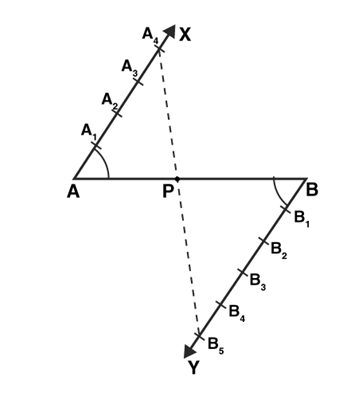(ii)Draw a line segment of length 7.6 cm and divide it in the ratio 5 : 8. Measure the two parts.

Solution:

Steps of construction:

1. Draw a line segment AB = 7.6 cm.

2. Draw a ray AX making an acute angle at A with AB.

3. Draw another ray BY parallel to AX making an acute angle. Make sure angle must be same as considered in step 2.

4. Taking A as center draw an arc cutting at A1 on the line.

Taking same radius consider A1 as a center and draw another arc cutting line at A2.

Repeat the same procedure and divide the line AX into 5 points A1, A2, A3, A4 and A5

In such a way, AA1 = A1A2 = A2A3 = A3A4 = A4A5

5. Similar to step 4,

Taking B as center draw an arc cutting at B1 on the line.

Taking same radius (set in step 4) consider B1 as a center and draw another arc cutting line at B2.

Repeat the same procedure and divide the line BY into 8 points in such a way that BB1 = B1B2 = B2B3 = B3B4 = B4B5 = B5B6 = B6B7 = B7B8

6. Join A5B8

7. Line A5B8 intersect AB at a point P in the ratio 5:8

8. Measurement: PB = 4.7 cm and AP = 2.9 cm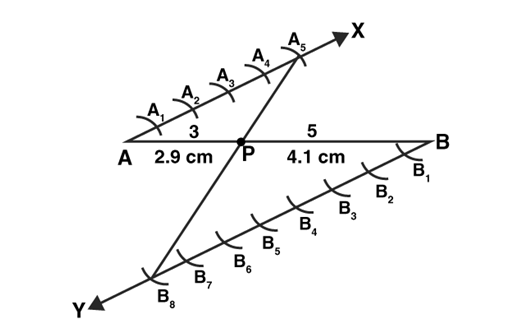Question 3: Construct a ∆PQR, in which PQ = 6 cm, QR = 7 cm and PR = 8 cm. Then, construct another triangle whose sides are 4/5 times the corresponding sides of ∆PQR.

Solution:

Steps of construction:

1. Draw a line segment PQ = 6 cm.

2. Draw an arc, using P as a center and radius = 8 cm

3. Draw another arc, using Q as a center and radius = 7 cm

4. Now, join PR and QR to get △PQR

5. Draw a ray PX by making an acute angle, angle QPX

6. Divide PX into 4 equal parts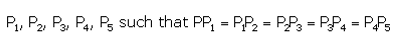7. Join P5Q

8. Draw a line P4Q’ which is parallel to P5Q

9. Similar to step 8, draw a line Q’R’ which is parallel to QR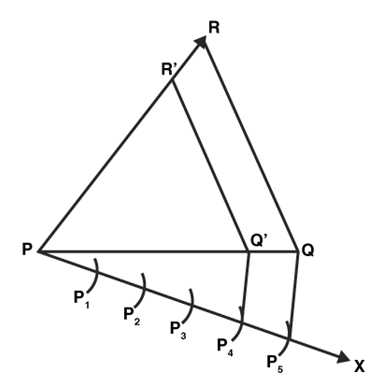Therefore, △PQ’R’ is the required triangle.

Question 4: Construct a triangle with sides 5 cm, 6 cm and 7 cm and then another triangle whose sides are 7/5 of the corresponding sides of first triangle.

Solution:

Steps of construction:

1. Draw a line segment BC = 5 cm.

2. Draw an arc, using B as a center and radius = 6 cm

3. Draw another arc, using C as a center and radius = 7 cm

4. Now, join AC and AB to get △ABC

5. Draw a ray BX by making an acute angle, angle CBX

6.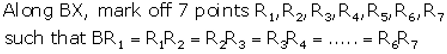7. Join R5C

8. Draw a line R7C’ which is parallel to R5C

9. Similar to step 8, draw a line C’A’ which is parallel to CA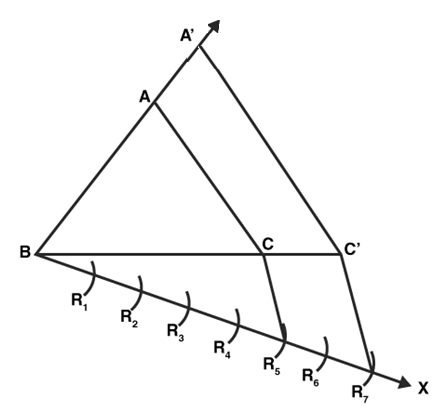Therefore,△A’BC’ is the required triangle.

Question 5: Construct a ∆ABC, with BC = 7 cm, ∠B = 60^o and AB = 6 cm. Construct another triangle whose sides are times the corresponding sides of ∆ABC.

Solution:

Steps of Construction:

1. Draw a triangle ABC with BC = 7 cm, ∠B = 60^0 and AB = 6 cm.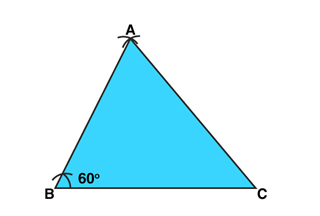2. Draw a ray BX making an acute angle with the line BC.

3. Divide BX into 4 equal arcs starting from B till B4. As shown in the below image.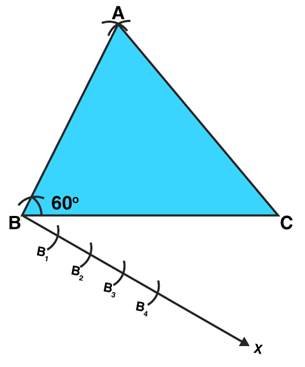4. Join B4C. Also draw a line from B3 parallel to B4C passing BC at C’.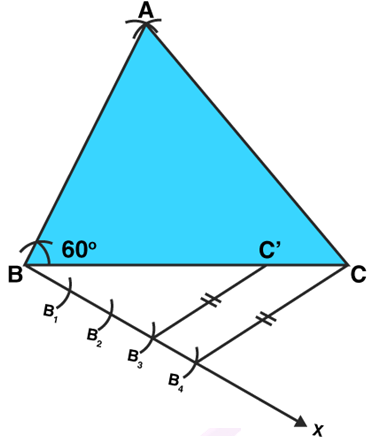5.Draw another line from C’ parallel to CA passing AB at A’.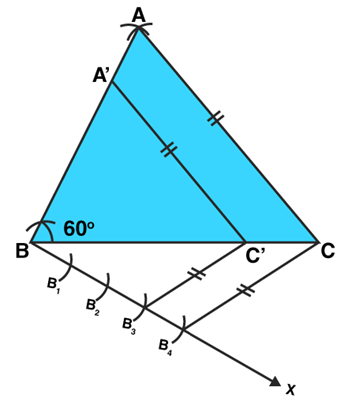Thus, △A’BC’ is required triangle.

Question 6: Construct a ∆ABC in which AB = 6 cm, ∠A = 30^o and ∠B = 60^o. Construct another ∆AB’C’ similar to ∆ABC with base AB’ = 8 cm.

Solution:

Construct a ∆ABC in which AB = 6 cm, ∠A = 30^o and ∠B = 60^o. Construct another ∆AB’C’ similar to ∆ABC with base AB’ = 8 cm.

1. Draw a line segment AB = 6cm and extend it to B’ such that AB’ = 8 cm.

2. Make an angle of 30° from A and angle of 60° from B. The point where these extended rays meet is C.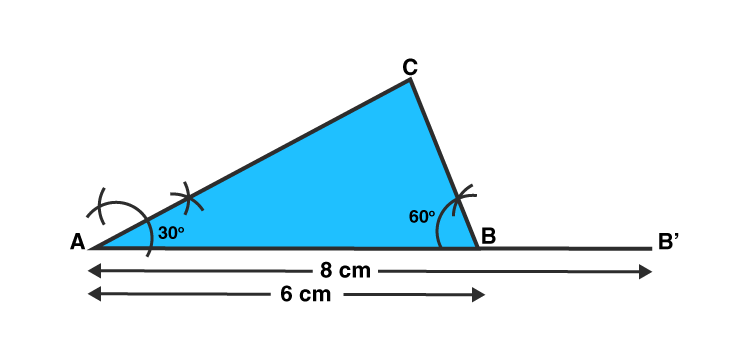3. Draw an angle of 60° from B’ (Similar triangles)

4. Extend line AC along C such that ray passing through point B’ Cut AX at C’.

5. AB’C’ is the required triangle.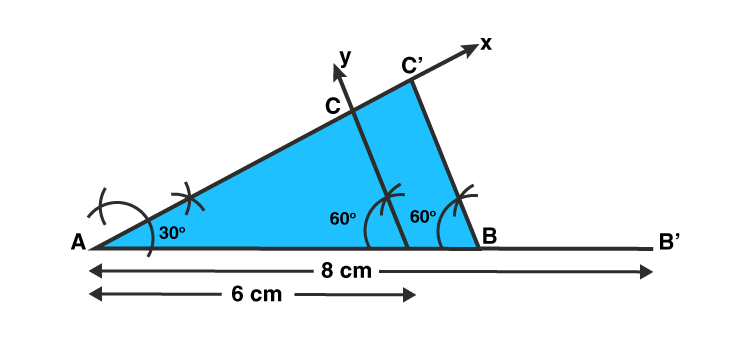Question 7: Construct a ∆ABC in which BC = 8 cm, ∠B = 45^0 and ∠C = 60^o. Construct another triangle similar to ∆ABC such that its sides are 3/5 of the corresponding sides of ∆ABC.

Solution:

Steps of Construction:

1. Draw a line segment BC = 8 cm.

2. Construct a triangle ABC based on given instructions.

3. Draw an arc below BC, by making an acute angle, angle CBZ

4. Divide BZ into 5 equal parts.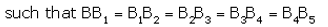5. Join B5C

6. Draw a line B3C’ which is parallel to B5C and join C’A’ which is parallel to CA.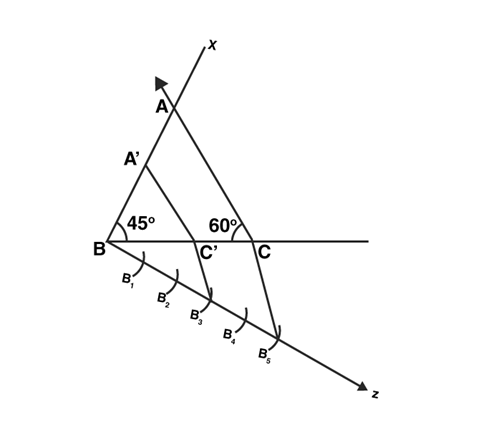Therefore, △A’BC’ is the required triangle.

Question 8: To construct a triangle similar to ∆ABC in which BC = 4.5 cm, ∠B = 45^o and ∠C = 60^o, using a scale factor of 3/7, BC will be divided in the ratio.

(a) 3 : 4 (b) 4 : 7 (c) 3 : 10 (d) 3 : 7

Solution:

BC will be divided in the ratio 3 : 4. Option (a) is correct.

Question 9: Construct an isosceles triangle whose base is 8 cm and altitude 4 cm and then another triangle whose sides are 1_1/2 (or 3/2) times the corresponding sides of the isosceles triangle.

Solution:

Steps of Construction:

1. Draw a line segment BC = 8 cm.

2. Draw a perpendicular bisector PQ of BC dividing BC at point M.

3. From QP cut off a distance MA = 4cm

4. Join AC and AB

5. Draw an arc below BC, by making an acute angle, angle CBX

6.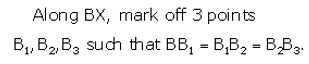7. Join B2C

8. Join B3C’which is parallel to B2C and Join A’C’ which is parallel to AC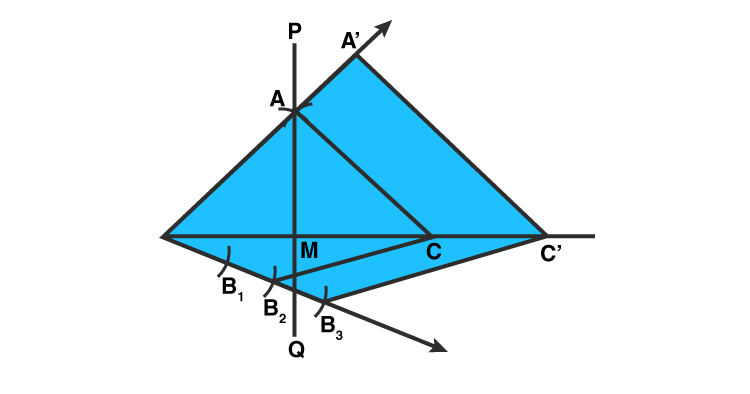Thus, △A’BC’ is required triangle.

Question 10: Draw a right triangle in which sides (other than hypotenuse) are of lengths 4 cm and 3 cm. Then, construct another triangle whose sides are 5/3 times the corresponding sides of the given triangle.

Solution:

Steps to construction:

1. Draw a line segment BC = 4 cm.

2. Construct, angle B = 90 degrees

3. Cut BA at the radius of 3 cm from BQ

4. Join AC (we have right triangle ABC)

5. Draw a ray BX and make an acute below BC, i.e. angle CBX

6.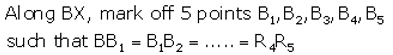7. Join B3C

8. Join B5C’ which is parallel to B3C and C’A’ which is parallel to AC.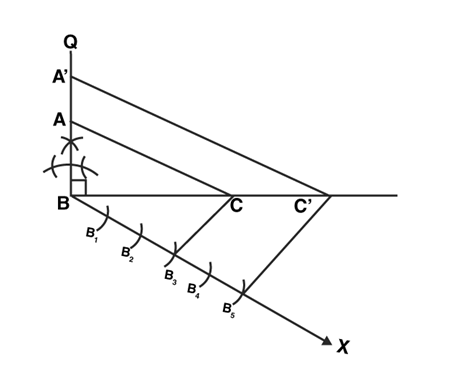## RS Aggarwal Solutions for Class 10 Maths Chapter 9 Constructions Exercise 9A

Class 10 Maths Chapter 9 Constructions Exercise 9A is based on the topics:

• Division of a line segment
• Construction of triangles